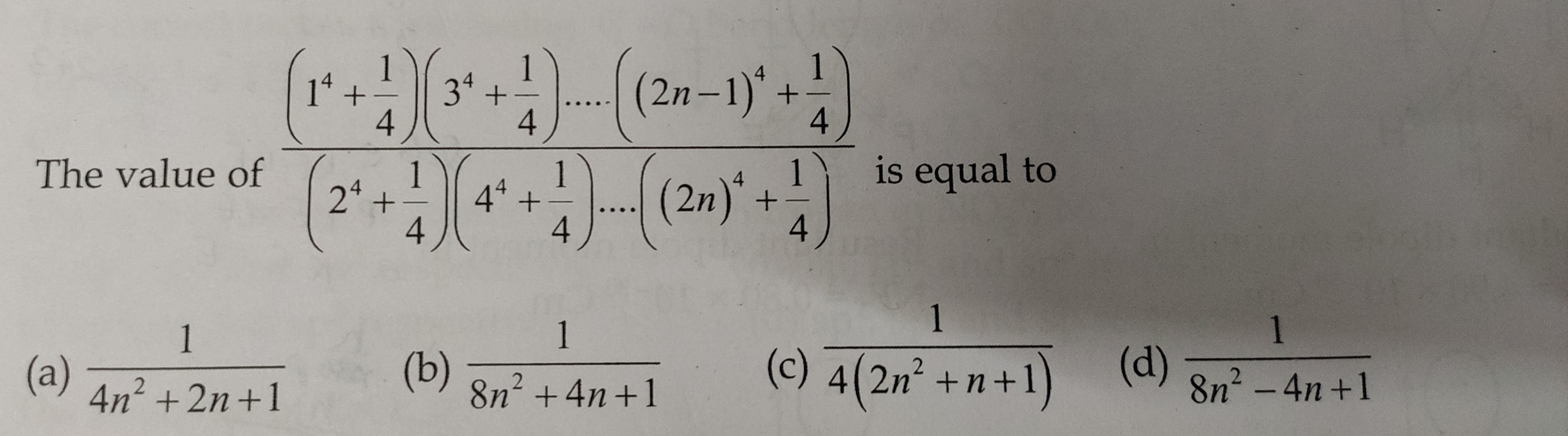# Sequence And Series

48 views

edited

The value of $\cfrac{(1^4+\frac14)(3^4+\frac14)...((2n-1)^4+\frac14)}{(2^4+\frac14)(4^4+\frac14)....((2n)^4+\frac14)}$ is equal toby (37.0k points)

Let P(n) = $\cfrac{(1^4+\frac14)(3^4+\frac14)...((2n-1)^4+\frac14)}{(2^4+\frac14)(4^4+\frac14)....((2n)^4+\frac14)}$

Put n = 1, we get P(1) = $\cfrac{1^4+\frac14}{2^4+\frac14}=\frac{1+\frac14}{16+\frac14}$ = $\cfrac{\frac54}{\frac{65}4}$

$\frac5{65}=\frac1{13}$

From option (a), $\frac{1}{4n^2+2n+1}$ at n = 1 = $\frac1{4+2+1}=\frac17$

Hence, (a) is wrong.

From option (b), $\frac1{8n^2+4n+1}$ at n = 1 = $\frac1{8 + 4 + 1}=\frac1{13}$

Hence, option(b) may be true.

From option (c), $\frac1{4(2n^2+n+1)}$ at n = 1 = $\frac1{4(2+1+1)}=\frac1{16}$

Hence, option (c) is wrong.

From option (d), $\frac1{4(8n^2-4n+1)}$ at n = 1 = $\frac{1}{8-4+1}=\frac15$

Hence, option(d) is wrong.

Only option (b) may be true.

$\therefore$ P(n) = $\frac1{8n^2+4n+1}$ which can be proved by mathematical induction.